# Solving Linear Inequalities Linear Inequality A linear inequality

• Slides: 14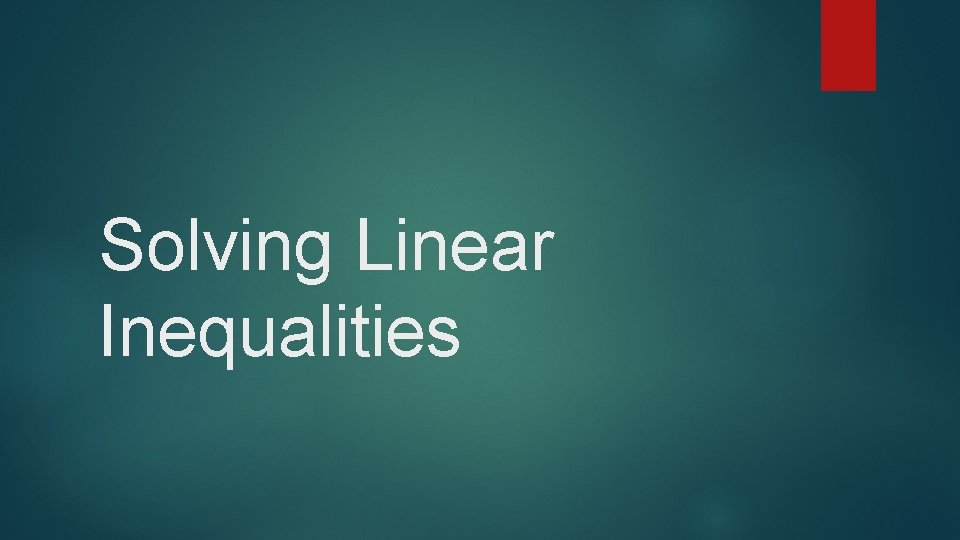Solving Linear InequalitiesLinear Inequality A linear inequality is similar to a linear equation, but the equal sign is replaced with an inequality signal. A solution of a linear inequality is any ordered pair that makes the inequality true.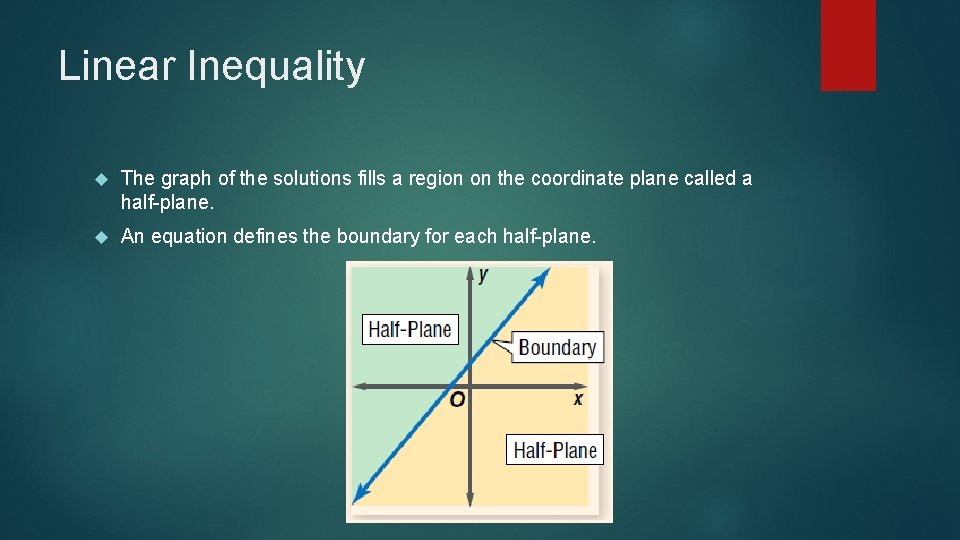Linear Inequality The graph of the solutions fills a region on the coordinate plane called a half-plane. An equation defines the boundary for each half-plane.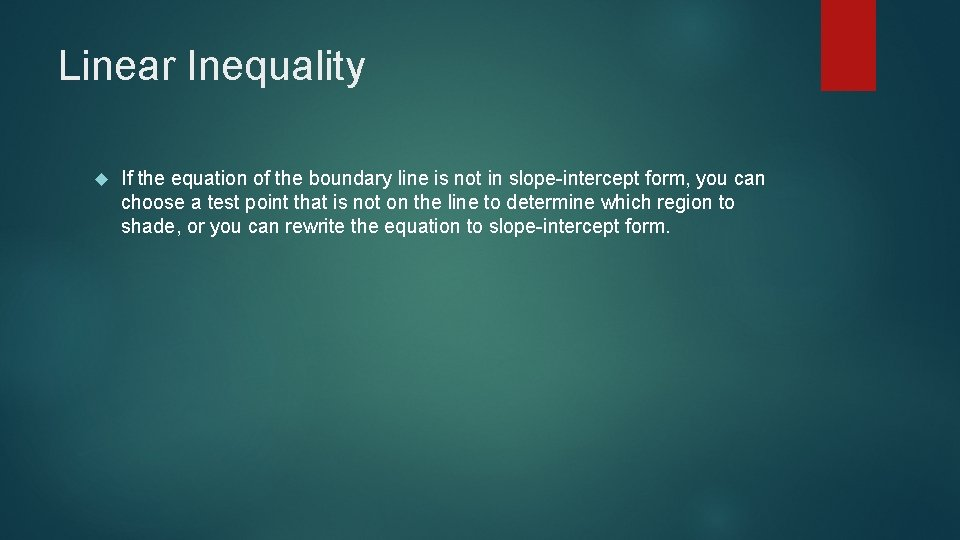Linear Inequality If the equation of the boundary line is not in slope-intercept form, you can choose a test point that is not on the line to determine which region to shade, or you can rewrite the equation to slope-intercept form.Linear InequalityExamples Graph y – 2 x ≤ -4Examples Graph y – 2 x ≤ -4 Method 1: Solve the equation for y. y – 2 x + 2 x ≤ -4 +2 x y ≤ 2 x -4 Graph y = 2 x – 4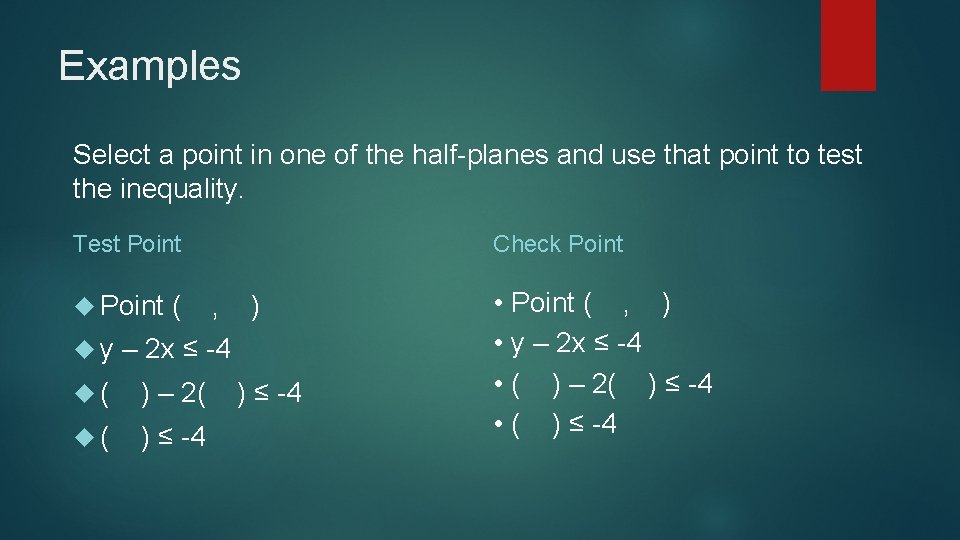Examples Select a point in one of the half-planes and use that point to test the inequality. Test Point Check Point ( , ) • Point ( , ) • y – 2 x ≤ -4 • ( ) – 2( ) ≤ -4 • ( ) ≤ -4 y – 2 x ≤ -4 ( ) – 2( ) ≤ -4ExamplesExamples Graph y – 2 x ≤ -4 Method 2: Find the intercepts of the line x-intercept: make y = 0; (0) – 2 x ≤ -4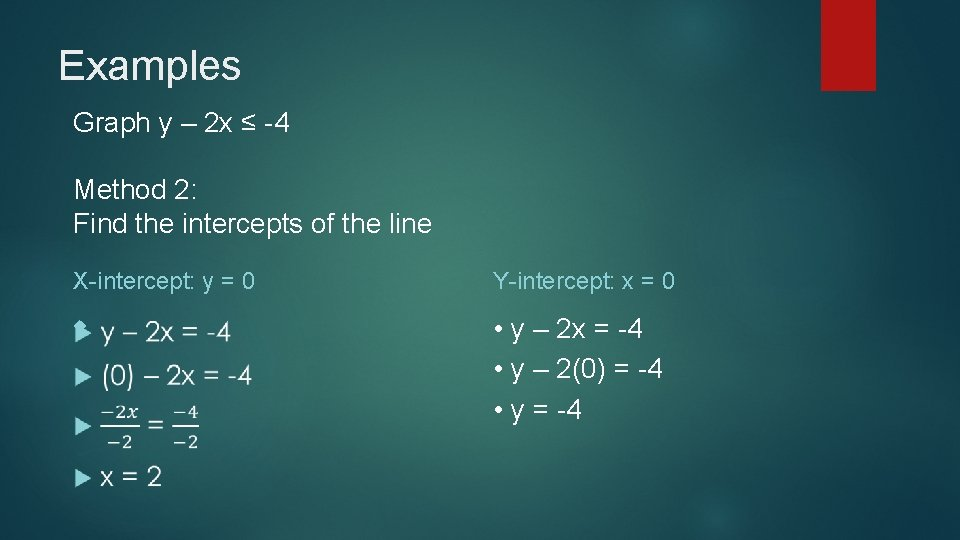Examples Graph y – 2 x ≤ -4 Method 2: Find the intercepts of the line X-intercept: y = 0 Y-intercept: x = 0 • y – 2 x = -4 • y – 2(0) = -4 • y = -4ExamplesExamples Select a point in one of the half-planes and use that point to test the inequality. Test Point Check Point ( , ) • Point ( , ) • y – 2 x ≤ -4 • ( ) – 2( ) ≤ -4 • ( ) ≤ -4 y – 2 x ≤ -4 ( ) – 2( ) ≤ -4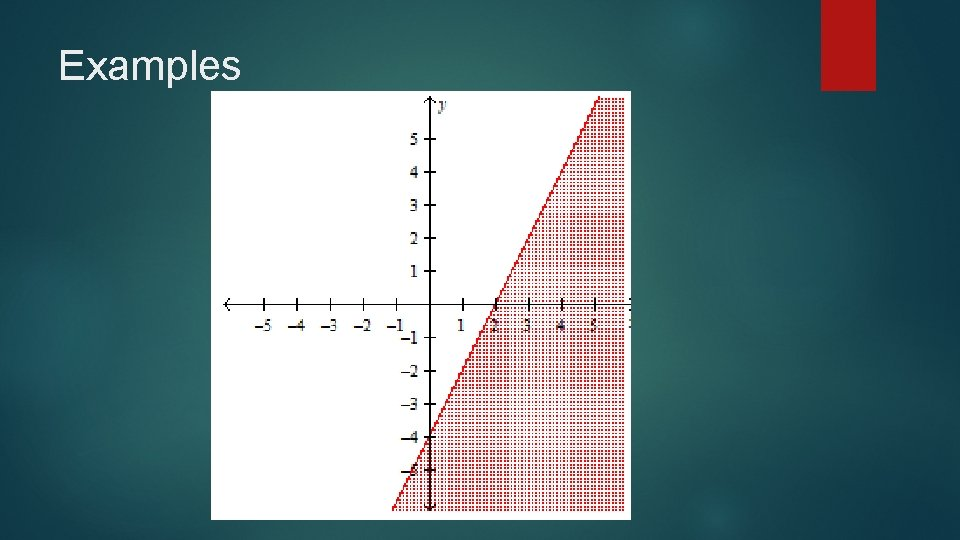Examples